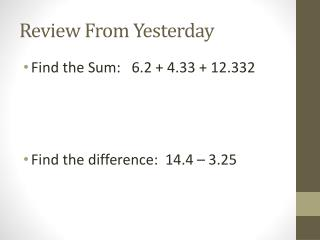DownloadDownload PresentationReview From Yesterday

# Review From Yesterday

Télécharger la présentation## Review From Yesterday

- - - - - - - - - - - - - - - - - - - - - - - - - - - E N D - - - - - - - - - - - - - - - - - - - - - - - - - - -
##### Presentation Transcript

1. Review From Yesterday • Find the Sum: 6.2 + 4.33 + 12.332 • Find the difference: 14.4 – 3.25

2. Review adding and subtracting • Find the Sum: 2.33 + 9.4 + 6.456 • Find the difference: 12.6 – 3.44

3. 3.5 Multiplying Decimals EQ: How do you solve problems involving the addition, subtraction, multiplication, and division of decimals? (SPI: 0606.2.3) /CC.6EE.2b

4. How do we multiply with decimals? 62 X 12 124 +620 744 Multiplying without decimals, multiply out like normal Below I multiplied using the same numbers, just added decimals, what do you notice? Can you identify a pattern? 62 X 1.2 124 +620 74.4 62 X .12 124 +620 7.44 6.2 X .12 124 +620 .744 .62 X .12 124 +620 .0744

5. Multiplying Decimals • To multiple numbers with decimals follow these three steps: • Multiply as if the factors were whole numbers • Count the total decimal places in the factors • Give the product the total decimal places of the factors

6. Step 1: Multiply as if the factors were whole numbers 8.5 X2.5 4 2 5 1 7 0 0 2 1 2 5 next…..

7. Step 2: Count the total decimal places in the factors 8.5 X2.5 4 2 5 1 7 0 0 2 1 2 5 next…..

8. Step 3: Give the product the total decimal places of the factors 8.5 X2.5 4 2 5 1 7 0 0 2 1 .2 5

9. 4 x 3.6 • What # should go on top? Why? • 3.55 x .05

10. .47 x 0.6 • 0.7 x 0.9

11. BOOK NOTE Multiplying Decimals You can use graph paper to model decimal multiplication. Here is a model of the product of0.7and0.3. 0.7 0.3 1 Because 21 of the 100 squares are shaded, 0.70.3 = 0.21. 1 Multiplying Decimals Words Multiply decimals as you would whole numbers. Then place the decimal point in the product. The number of decimal places in the product is equal to the sum of the number of decimal places in the factors. 0.70.3 = 0.21 Numbers

12. .31 x .7 = .217 .9 x .03 = .027 2 x .006 = .012 .66 x 2.52 = 1.6632 Showdown

13. How well do you understand multiplying decimals? . I fully understand I don’t understand I nearly understand

14. Think About it…… • 12 ÷ 6 = 2 • 12 ÷ 0.6 = 20 • What happens when we divide? What do you notice about the quotient? • 12 x 6 = 72 • 12 x 0.6 = ?? • What do you think the product of 12 x 0.6 is going to be? Why do you think this?